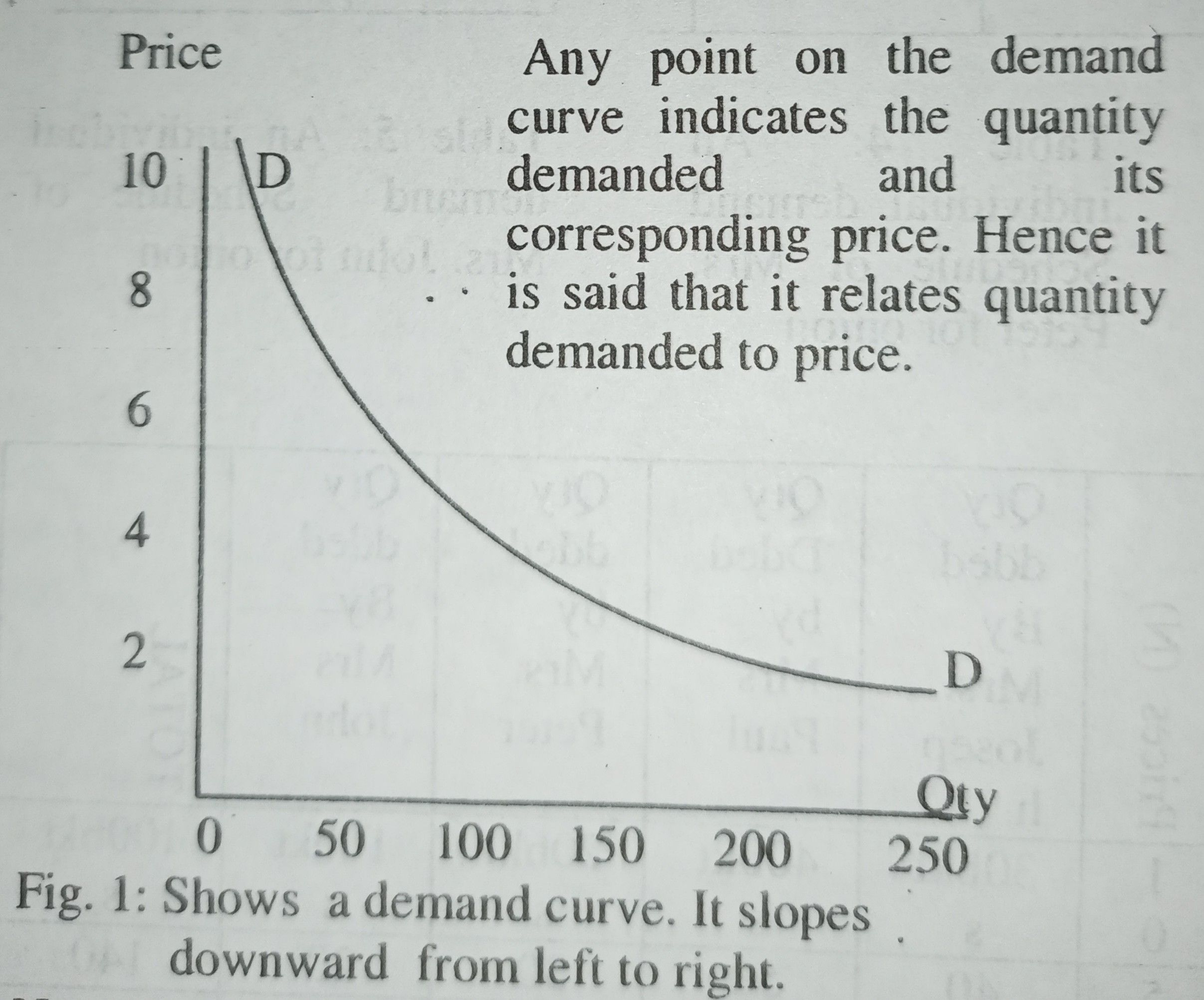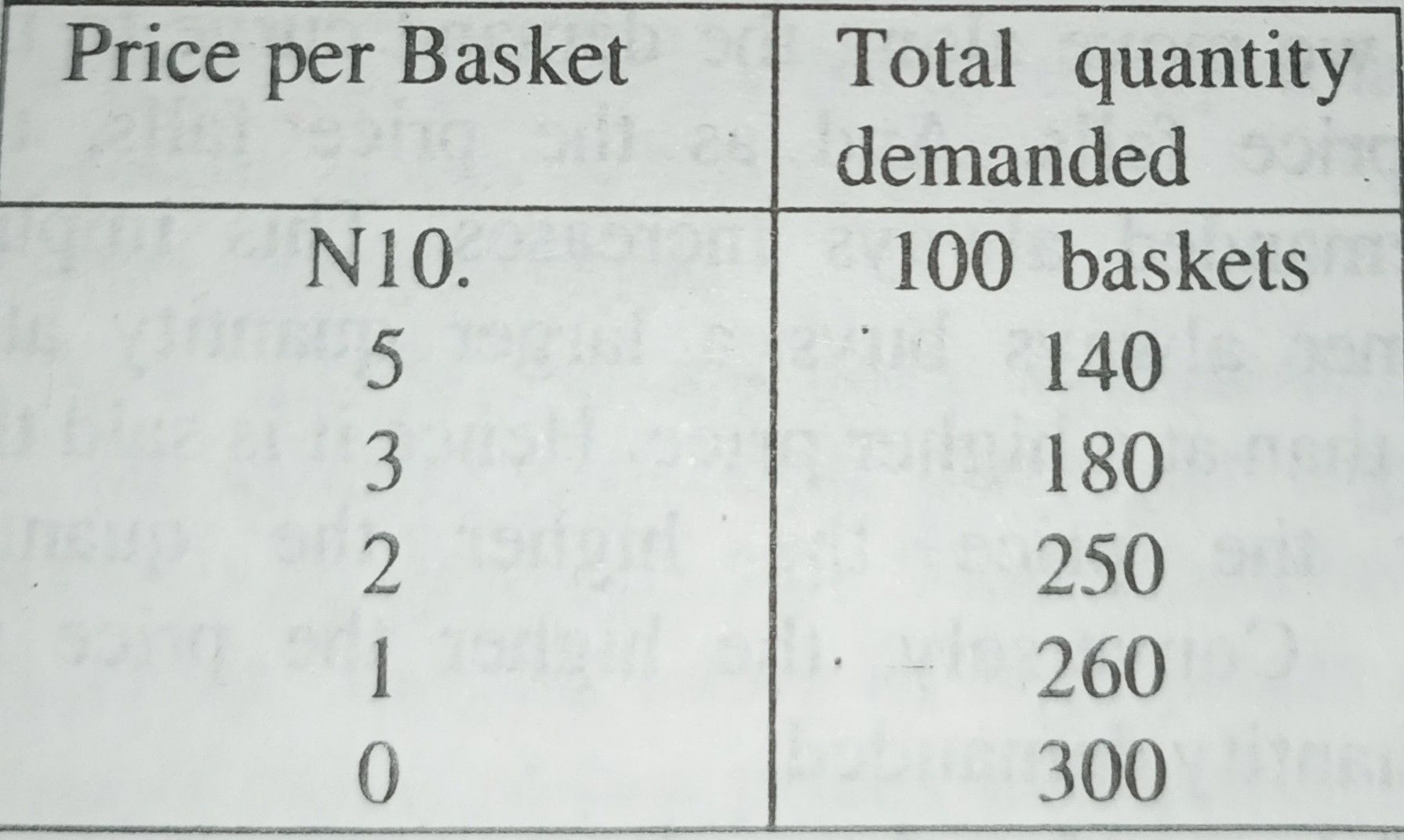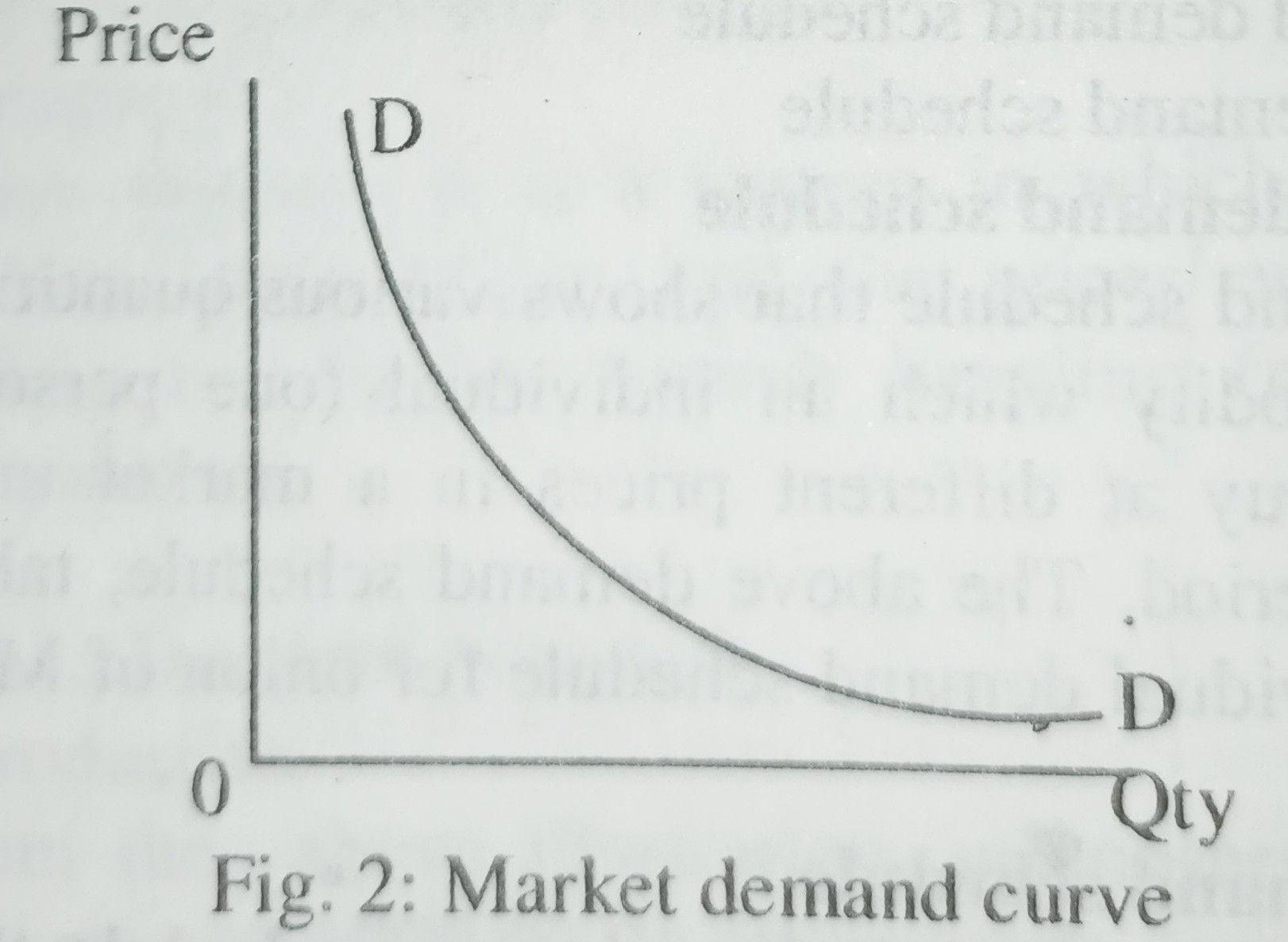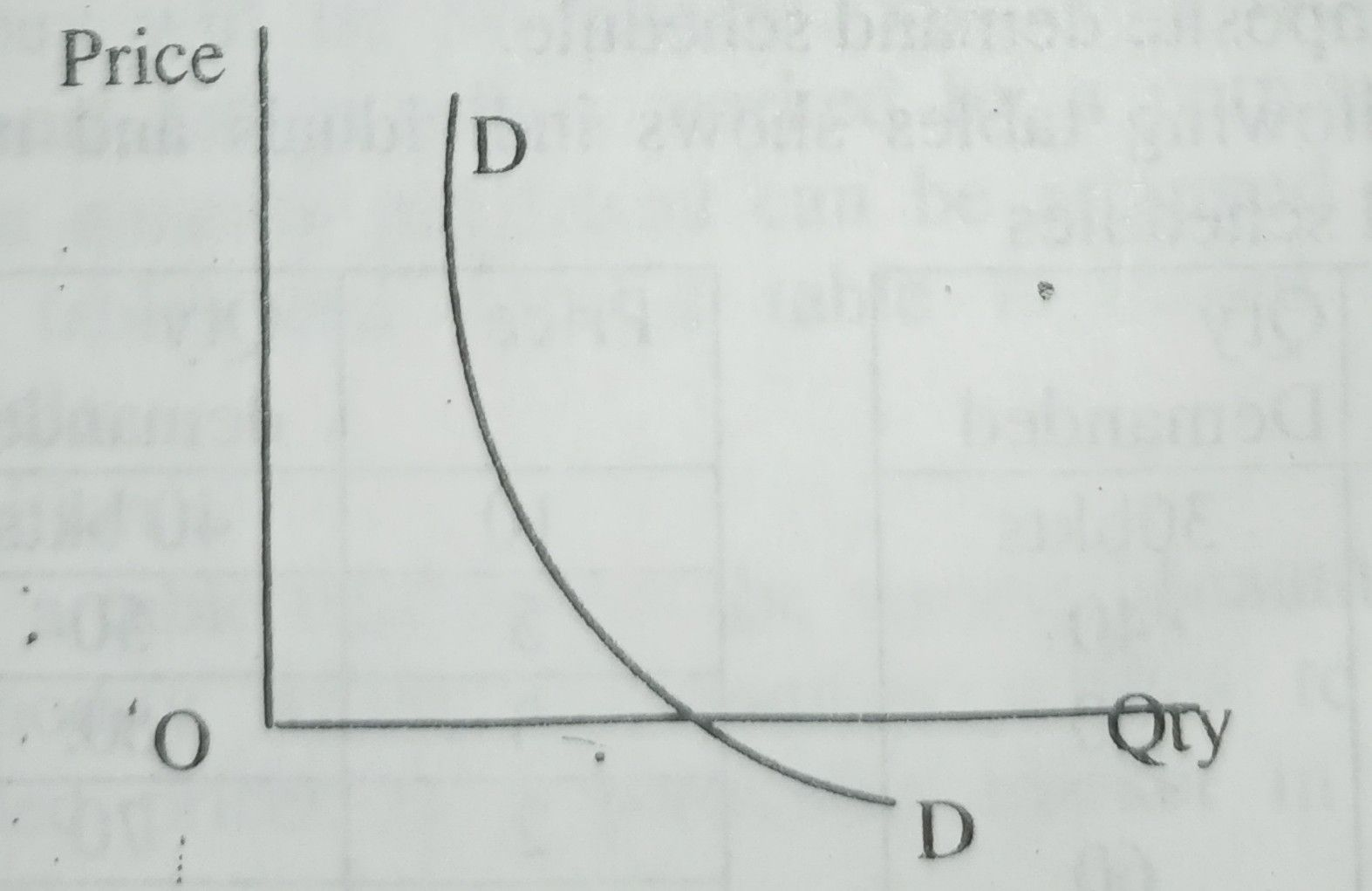Home » DEMAND CURVE

# DEMAND CURVE• It is a curve that relates different quantities of a commodity demanded to their various prices. That is, it shows relationship between quantities demanded and their prices. Briefly, it is a curve that relates quantity demanded to each price.
• Demand curve is a graphical representation of the demand schedule. That is, it is a graphical representation of the quantity demanded at each price. Thus it shows at a glance the quantity demanded at each price.
• Demand curve is a graphical relation showing different quantities of a commodity which a consumer wishes to buy at different prices.In a normal demand schedule and curve, quantity demanded is negatively or inversely related to price.

Increase in price causes a fall in quantity demanded. Thus a normal demand curve is negatively sloped.

A demand curve is concave to the point of origin.

Slope of demand curve

The demand curve slopes downward from left to the right. Its slope enables us to make true statement about the economic behaviour of the consumer.

As we move along the demand curve to the right, the price falls. And as the price falls, the quantity demanded always increases. This implies that consumer always buys a larger quantity at a lower price than at a higher price. Hence it is said that ‘the lower the price the higher the quantity demanded’. Conversely, the higher the price the lower the quantity demanded.

First law of demand and supply.

The slope of the above demand curve reaffirms the economic hypothesis (assumption or statement) that:

‘The lower the price, the higher the quantity that would be demanded’.

The above statement is the first law of demand and supply. The law implies that a price is related to quantity demanded.

Types of demand curve.

The following are the major types of demand curve

1) Individual demand curve

It is a curve that relates the quantity of a commodity demanded by an individual (one person) to the price. It shows the quantity demanded by only one person at each price. Thus the data (figures) in table 2 are used to plot (draw) individual demand curve as shown in figure 1.

2) Market demand curve

It is a curve that relates the total quantity of a commodity demanded by all consumers in a market to each price. It is also referred to as the horizontal summation (addition) of all individual demand curves. And any point on it shows the total quantity demanded by all buyers in a market at each price. The data in table below are used to plot the market demand curve as shown in figure 2.Market demand curve is drawn under the assumption that taste, income and other prices are held constant or ignored.

The individual demand curve intersects (cuts) the X axis when the price is zero. While the market demand curve intersects the X axis at the total quantity (summation or addition) of individual demand when the price is zero. This is shown in figure 3 below.